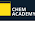### Equation of Charge Neutrality

Consider a semiconductor material which is doped with both type of impurities, donor as well as acceptor. The concentration of donor impurity is and that of acceptor impurity is  NA.
Each donor atom donates one electron and as it losses electron it becomes positively charged ion, due to the process of ionization. Thus assuming ionization of all the impurity atoms, the concentration of positive ions generated due to donor impurity becomes ND. There are positive charge carriers i.e. holes present in the material whose concentration is p. This the concentration of total positive charge in the material is given by,
Total positive charge concentration =  ND + p                                ..............(1)
The acceptor impurity atom when added to the material accepts an electron and becomes negatively charged ion. Assuming ionization of all the impurity atoms, the concentration of negative ions generated due to acceptor impurity is NA. There are negative charge carriers i.e. electron present in the material whose concentration is n. Thus the concentration of total negative charge in the material is given by,
Total negative charge concentration = NA+ n                                  .............. (2)
The material as a whole is always electrically neutral hence the total positive charge concentration in the material must be equal to the total negative charge concentration in the material. Hence equating (1) and (2) we can write,
ND + p = NA + n                                                                         .................... (3)
The equation (3) is called the equation of charge neutrality.
Notes
1. The equation is important when a semiconductor is doped with both the types of impurities, donor as well as acceptor.
2. The equation is efficient in obtaining the actual concentration of electrons and holes in a material which is doped with both the types of impurities.
3. The equation helps to determine whether the material doped with both the types of impurities will behave as n-type or p-type.

1.